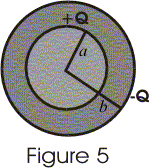# Spherical capacitor

## Spherical capacitor

• A spherical capacitor consists of a solid or hollow spherical conductor of radius a , surrounded by another hollow concentric spherical of radius b shown below in figure 5• Let +Q be the charge given to the inner sphere and -Q be the charge given to the outer sphere.
• The field at any point between conductors is same as that of point charge Q at the origin and charge on outer shell does not contribute to the field inside it.
• Thus electric field between conductors is $$E=\frac{Q}{2\pi \epsilon _{0}r^{2}}$$
• Potential difference between two conductors is
$V=V_a -V_b$
$=- \int E.dr$
where limits of integration goes from a to b.
On integrating we get potential difference between to conductors as
$$V=\frac{Q(b-a)}{4\pi \epsilon _{0}ba}$$
• Now , capacitance of spherical conductor is
$C= \frac {Q}{V}$
or,
$C=\frac{4\pi \epsilon _{0}ba}{(b-a)}$ ----(1)
• again if radius of outer conductor approaches to infinity then from equation 6 we have
$C=4 \pi \epsilon _{0} a$ ----(2)
• Equation 2 gives the capacitance of single isolated sphere of radius a.
• Thus capacitance of isolated spherical conductor is proportional to its radius.

## Spherical capacitor when inner sphere is earthed

• If a positive charge of Q coulombs is given to the outer sphere B, it will distribute itself over both its inner and outer surfaces.
• Let the charges of $Q_1$ and $Q_2$ coulombs be at the inner and outer surfaces respectively of sphere B where $Q = Q_1 +Q_2$,
• The charge + $Q_1$ on the inner surface of outer sphere B will induce a charge of -$Q_1$ coulombs on the outer surface of inner sphere A and + $Q_1$ coulombs on the inner surface of sphere A, which will go to earth.
• Now there are two capacitors connected in parallel.
(i) One capacitor consists outer surface of sphere B and earth having capacitance $C_1 = 4 \pi \epsilon _0 b$ farads
(ii) Second capacitor consisting of inner surface of outer sphere B and the outer surface of inner sphere A having capacitance
$C_2=\frac{4\pi \epsilon _{0}ba}{(b-a)}$
• Final Capacitance
$C=C_1+C_2=4 \pi \epsilon _0 b + \frac{4\pi \epsilon _{0}ba}{(b-a)}= \frac{4\pi \epsilon _{0}b^2}{(b-a)}$